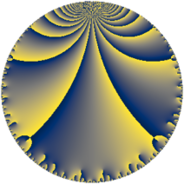# Properties

 Label 29.6.dLevel $29$ Weight $6$ Character orbit 29.d Rep. character $\chi_{29}(7,\cdot)$ Character field $\Q(\zeta_{7})$ Dimension $66$ Newform subspaces $1$ Sturm bound $15$ Trace bound $0$

# Related objects

## Defining parameters

 Level: $$N$$ $$=$$ $$29$$ Weight: $$k$$ $$=$$ $$6$$ Character orbit: $$[\chi]$$ $$=$$ 29.d (of order $$7$$ and degree $$6$$) Character conductor: $$\operatorname{cond}(\chi)$$ $$=$$ $$29$$ Character field: $$\Q(\zeta_{7})$$ Newform subspaces: $$1$$ Sturm bound: $$15$$ Trace bound: $$0$$

## Dimensions

The following table gives the dimensions of various subspaces of $$M_{6}(29, [\chi])$$.

Total New Old
Modular forms 78 78 0
Cusp forms 66 66 0
Eisenstein series 12 12 0

## Trace form

 $$66q - 11q^{2} - 5q^{3} - 171q^{4} + 29q^{5} + 445q^{6} + 17q^{7} + 1046q^{8} - 732q^{9} + O(q^{10})$$ $$66q - 11q^{2} - 5q^{3} - 171q^{4} + 29q^{5} + 445q^{6} + 17q^{7} + 1046q^{8} - 732q^{9} - 141q^{10} + 1125q^{11} - 248q^{12} - 1789q^{13} + 1233q^{14} + 1061q^{15} + 2629q^{16} + 676q^{17} - 10896q^{18} - 1903q^{19} + 4081q^{20} - 439q^{21} - 2980q^{22} + 9945q^{23} + 10741q^{24} - 22114q^{25} - 1641q^{26} - 4427q^{27} + 7508q^{28} - 13100q^{29} - 40018q^{30} + 8541q^{31} + 18996q^{32} + 20725q^{33} - 8839q^{34} + 14127q^{35} + 70172q^{36} + 3791q^{37} + 36322q^{38} + 25843q^{39} - 72621q^{40} - 18720q^{41} - 72614q^{42} - 10419q^{43} + 35796q^{44} + 23163q^{45} + 15848q^{46} - 40549q^{47} - 4194q^{48} + 19808q^{49} + 201932q^{50} + 51998q^{51} + 8330q^{52} - 72275q^{53} - 197108q^{54} + 42183q^{55} + 31599q^{56} - 40162q^{57} - 258031q^{58} - 333512q^{59} + 26920q^{60} - 175281q^{61} + 213745q^{62} + 354423q^{63} - 19790q^{64} - 79593q^{65} + 103783q^{66} + 135179q^{67} + 334644q^{68} + 73673q^{69} - 511678q^{70} - 187563q^{71} - 17551q^{72} - 56675q^{73} + 159980q^{74} + 538208q^{75} - 215041q^{76} + 106099q^{77} + 37481q^{78} + 273775q^{79} + 571652q^{80} - 61356q^{81} - 37092q^{82} - 237831q^{83} + 127523q^{84} - 148844q^{85} - 348400q^{86} - 110493q^{87} - 985638q^{88} + 74239q^{89} - 630714q^{90} + 100309q^{91} + 132694q^{92} - 307921q^{93} + 206074q^{94} + 37965q^{95} + 687094q^{96} + 136055q^{97} - 115704q^{98} + 438608q^{99} + O(q^{100})$$

## Decomposition of $$S_{6}^{\mathrm{new}}(29, [\chi])$$ into newform subspaces

Label Dim. $$A$$ Field CM Traces $q$-expansion
$$a_2$$ $$a_3$$ $$a_5$$ $$a_7$$
29.6.d.a $$66$$ $$4.651$$ None $$-11$$ $$-5$$ $$29$$ $$17$$